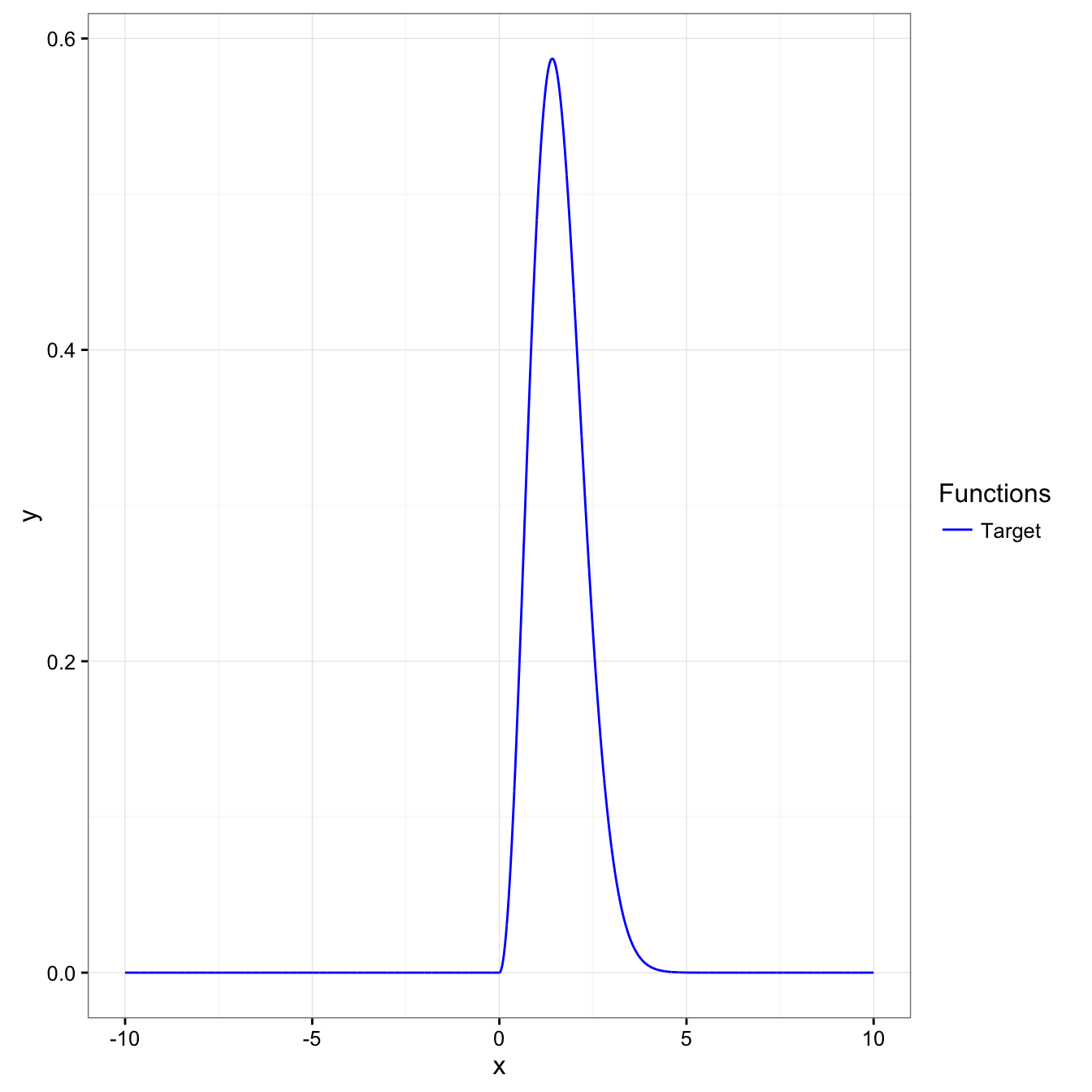library(magrittr)
library(ggplot2)

## Examine the target distribution

$$f(x) \propto \exp \left(- \frac{(x - \mu)^2}{2\sigma^2}\right)x^2 I(x \ge 0)$$

For simplicity, $$\mu = 0, \sigma^2 = 1$$ are used. The normalizing constant is the inverse of $. ## Target density constructor (normalized) construct_target_density <- function(mu, sigma) { function(x) { ifelse(x >= 0, exp(-(x - mu)^2 / (2 * sigma^2)) * x^2, 0) / (sqrt(2*pi) / 2) } } ## Simple case with mu = 0, sigma = 1 target_density0 <- construct_target_density(mu = 0, sigma = 1) ## Examine visually to examine peak height g1 <- ggplot(data = data.frame(x = 0), mapping = aes(x = x)) + geom_path(stat = "function", fun = target_density0, n = 10001, mapping = aes(color = "1")) + scale_x_continuous(limits = c(-10,10)) + scale_color_manual(name = "Functions", values = c("blue", "red", "green"), # Color specification labels = c("Target", "N(0,1^2)", "10*N(0,3^2)")) + theme_bw() + theme(legend.key = element_blank()) print(g1)## Implement Metropolis-Hasting sampler The proposal distribution proposing a move to $$x^{(j+1)}$$ from the current value $$x^{(j)}$$ should be a distribution centered at $$x^{(j+1)}$$ and should respect the nonnegative support. Thus, we will use $$Expo\left(- \frac{1}{x^{(j)}}\right)$$ as the proposal distribution. Importantly, this proposal distribution is not symmetric around the current value, therefore, $$q(x^{(j+1 )} | x^{(j)}) = q(x^{(j)} | x^{(j+1)})$$ does not hold in general. Thus, the acceptance probability is $$\alpha(x^{(j+1)} | x^{(j)}) = \min \left(1, \frac{\pi(x^{(j+1)}) q(x^{(j)} | x^{(j+1)})}{ \pi(x^{(j)}) q(x^{(j+1)} | x^{(j)})}\right)$$. MHSampling <- function(target_density, proposal_density, proposal_generator, init, R) { ## Initialize a vector x <- numeric(length = R) ## Acceptance indicator a <- logical(length = R) ## Initial value x <- init for (r in seq_len(R)[-1]) { ## Obtain a proposed value conditioning on the previous value proposal <- proposal_generator(x[r-1]) ## Evaluate both densities at the proposed value target_at_proposal <- target_density(proposal) target_at_previous <- target_density(x[r-1]) ## This is assured to be [0,1] acceptance_ratio <- min((target_at_proposal * proposal_density(x[r-1], proposal)) / (target_at_previous * proposal_density(proposal, x[r-1])), 1) if (runif(n = 1) <= acceptance_ratio) { ## If U(0,1) is less than or equal to acceptance ratio ## Make a move x[r] <- proposal a[r] <- TRUE } else { ## Otherwise, don't move x[r] <- x[r-1] } } data.frame(x = x, a = a) } out1 <- MHSampling(target_density = target_density0, proposal_density = function(x_next, x) {dexp(x = x_next, rate = 1 / x)}, proposal_generator = function(x) {rexp(n = 1, rate = 1 / x)}, init = 3, R = 10^5) out1$i <- seq_along(out1$x) ## Acceptance ratio mean(out1$a)
##  0.40905
## Plot of chain
ggplot(data = out1, mapping = aes(x = i, y = x)) +
geom_point(alpha = 1 / 5) +
geom_line(alpha = 1 / 5) +
theme_bw() + theme(legend.key = element_blank())`